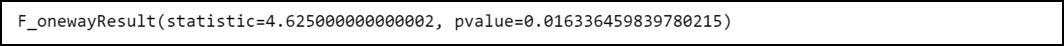Open in App
Not now

# How to Perform a One-Way ANOVA in Python

• Difficulty Level : Easy
• Last Updated : 04 Jul, 2022

One-Way ANOVA in Python: One-way ANOVA (also known as “analysis of variance”) is a test that is used to find out whether there exists a statistically significant difference between the mean values of more than one group.

## Hypothesis involved:

A one-way ANOVA has the below given null and alternative hypotheses:

• H0 (null hypothesis): μ1 = μ2 = μ3 = … = μk (It implies that the means of all the population are equal)
• H1 (null hypothesis): It states that there will be at least one population mean that differs from the rest

Statement:

Researchers took 20 cars of the same to take part in a study. These cars are randomly doped with one of the four-engine oils and allowed to run freely for 100 kilometers each. At the end of the journey, the performance of each of the cars is noted. Before proceeding further we need to install the SciPy library in our system. You can install this library by using the below command in the terminal:

`pip3 install scipy`

## Stepwise Implementation

Conducting a One-Way ANOVA test in Python is a step by step process and these steps are explained below:

Step 1: Creating data groups.

The very first step is to create three arrays that will keep the information of cars when d

## Python3

 `# Performance when each of the engine ` `# oil is applied` `performance1 ``=` `[``89``, ``89``, ``88``, ``78``, ``79``]` `performance2 ``=` `[``93``, ``92``, ``94``, ``89``, ``88``]` `performance3 ``=` `[``89``, ``88``, ``89``, ``93``, ``90``]` `performance4 ``=` `[``81``, ``78``, ``81``, ``92``, ``82``]`

Step 2: Conduct the one-way ANOVA:

Python provides us f_oneway() function from SciPy library using which we can conduct the One-Way ANOVA.

## Python3

 `# Importing library` `from` `scipy.stats ``import` `f_oneway`   `# Performance when each of the engine ` `# oil is applied` `performance1 ``=` `[``89``, ``89``, ``88``, ``78``, ``79``]` `performance2 ``=` `[``93``, ``92``, ``94``, ``89``, ``88``]` `performance3 ``=` `[``89``, ``88``, ``89``, ``93``, ``90``]` `performance4 ``=` `[``81``, ``78``, ``81``, ``92``, ``82``]`   `# Conduct the one-way ANOVA` `f_oneway(performance1, performance2, performance3, performance4)`

Output:Output

Step 3: Analyse the result:

The F statistic and p-value turn out to be equal to 4.625 and 0.016336498 respectively. Since the p-value is less than 0.05 hence we would reject the null hypothesis. This implies that we have sufficient proof to say that there exists a difference in the performance among four different engine oils.

My Personal Notes arrow_drop_up
Related Articles